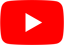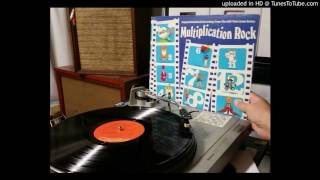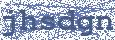Figure Eight
Blossom Dearie

## BLOSSOM DEARIE

Figure Eight Lyrics

Figure eight is double four
Figure four is half of eight
If you skate, you would be great
If you could make a figure eight
That's a circle that turns 'round upon itself

One times eight is two times four
Four times four is two times eight
If you skate upon thin ice
You'd be wise if you thought twice
Before you made another single move

One times eight is eight
Two times eight is 16
Three times eight is 24
Four times eight is 32
And five times eight is 40, you know

Six times eight is 48
Seven times eight is 56
Eight times eight is 64
Nine times eight is 72
And ten times eight is 80, that's true
Eleven times eight is 88
And twelve times eight is 96
Now, here's a chance to get off
'Cause twelve times eight is the same as
Ten times eight plus two times eight
80 plus 16
96

One times eight is eight
Two times eight is 16
Three times eight is 24
Four times eight is 32
And five times eight is 40, you know

Figure eight is double four
Figure four is half of eight
If you skate, you would be great
If you could make a figure eight
That's a circle that turns 'round upon itself
Place it on its side and it's a symbol meaning infinityHottest Lyrics with Videos
73146c3afa2f500cff1143ab9658c893